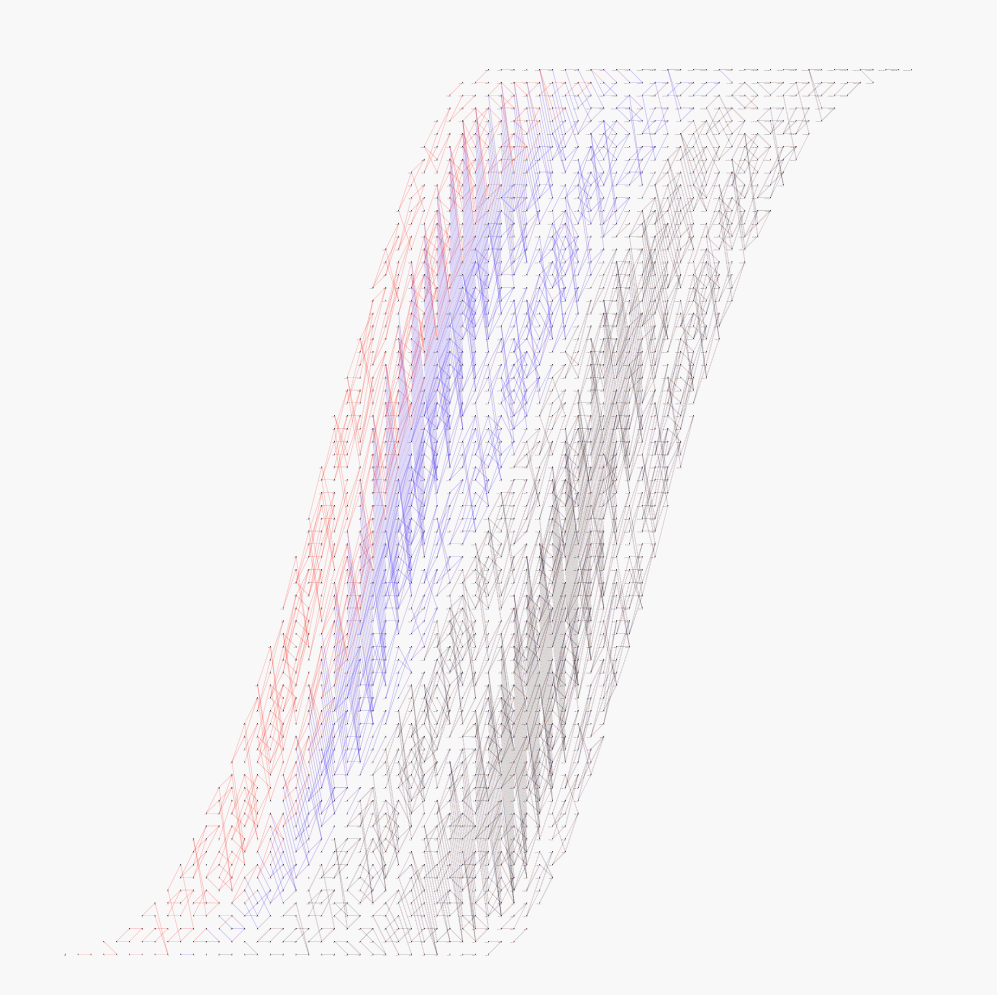The Cohomology of SO(n)The cohomology of the special orthogonal group SO(n) with integer coefficients, particularly its cup product structure, turns out to be rather complicated for so central and classical an object as SO(n) is. In principle the structure of the integer cohomology can be deduced from the simpler mod 2 cohomology using Bockstein homomorphisms, as described in Section 3E of my algebraic topology book where the special cases n=5 and n=7 are worked out. There is an algorithm for computing the Bocksteins which can be carried out by hand for small values of n, but computer assistance makes this much more practicable for larger n. M A Agosto and J J Perez have written a Mathematica program to do this, and the output for n=5,...,12 is shown in the following pdf files:

For an explanation of how to read the diagrams see either Example 3E.7 of my book or these brief comments. Note that it is possible to magnify the more complicated diagrams by repeatedly zooming in with your pdf viewer to make all the fine detail legible, including the labels on the dots. For more information about the Mathematica program see this page.

Here are some more diagrams showing several values of n at once using different colors:

The whole collection of diagrams with my introductory comments is also available as a single pdf file.